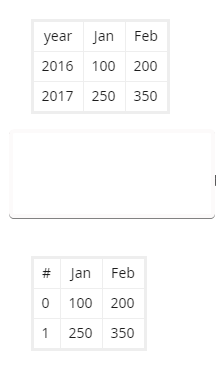# Beginner Question - Bokeh Datatable Pandas Index

New to Bokeh and Python here. I’ve tried searching and am struggling to find the exact thing. I keep thinking it is a simple issue with index number/value but I can’t seem to solve it.

I’ve put a couple charts on a page and am wanting to add a Data Table widget as well. I can get the datatable on there and everything is ALMOST right.

I’m doing a year over year table, so I’m pivoting my data because in the end I want to see a row for each year, then 1 column for each month of the year.

If I pivot this and look at the Pandas dataframe, everything looks great. (top example in picture)If I put this in a datatable widget though, the months and actuals all line up great…but the year is gone and replaced with what I assume is just a row number/index as shown in the bottom example in picture.

What would be the way I’d go about displaying the year as well? I know there’s something simple here I’m missing…just can’t figure out how I need to reshape.

``````def make_three_year_table(selected_account):
df = selected_account[['account', 'year', 'month', 'actual']]
df = df.pivot(index='year', columns='month', values='actual')
df = df[['Jan', 'Feb', 'Mar', 'Apr', 'May', 'Jun', 'Jul', 'Aug', 'Sep', 'Oct',
'Nov', 'Dec']]
columns = [TableColumn(field=Ci, title=Ci) for Ci in df.columns]
data_table = DataTable(columns=columns, source=ColumnDataSource(df))
return data_table``````
1 Like

I guess removing index from DataTable should work.

Try this

`data_table.index_position = None`

Another method would be to reset index in data frame like `df = df.reset_index()` and use the index as a column instead. Let us know which works best for you.

1 Like

Thanks!

Took some tweaking but a mixture of the reset_index idea and me stepping back and trying to manually do things I could see what was causing the hiccup.

It must be loop to define “columns” that was causing the issue, which makes sense if it was an index. If I manually identify the columns everything works and lines up like I’d expect.

``````def make_account_data_table(account_df):
df = account_df[['year', 'month', 'actual']]
df = df.pivot_table(index="year", columns="month", values="actual")
columns = [
TableColumn(field="year", title="Year"),
TableColumn(field="Jan", title="Jan"),
TableColumn(field="Feb", title="Feb"),
TableColumn(field="Mar", title="Mar"),
TableColumn(field="Apr", title="Apr"),
TableColumn(field="May", title="May"),
TableColumn(field="Jun", title="Jun"),
TableColumn(field="Jul", title="Jul"),
TableColumn(field="Aug", title="Aug"),
TableColumn(field="Sep", title="Sep"),
TableColumn(field="Oct", title="Oct"),
TableColumn(field="Nov", title="Nov"),
TableColumn(field="Dec", title="Dec")]
# columns = [TableColumn(field=Ci, title=Ci) for Ci in df.columns]
data_table = DataTable(columns=columns, source=ColumnDataSource(df))
data_table.index_position = None
data_table.width = 800
return data_table
``````

Thanks for the help!

Yes I think this exactly correct. Setting `index_position = None` hides the automatic table row index column that the table widget can show. When you do:

``````columns = [TableColumn(field=Ci, title=Ci) for Ci in df.columns]
``````

You explicitly adding columns that will always be displayed. So if you happen to explicitly add a dataframe index in that loop, it will get displayed (`index_position` has nothing to say about that, this is just another user-provided column).

2 Likes